Hello Guest it is October 04, 2023, 10:50:24 PM

###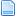Author Topic: can any one chech this code  (Read 4755 times)

0 Members and 1 Guest are viewing this topic.

####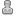swetha22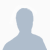•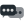9##### can any one chech this code
« on: January 02, 2009, 01:10:01 PM »
i was trying to get angle of x,y .Please check the following code and tell me what i am doing wrong .
assumed result of xangle is 45 and i am getting 37.20.
Dim x1
Dim x2
Dim y1
Dim y2

Dim x0
Dim y0
Dim hy
Dim aasine
Dim xan

x1 = -50
x2 = 0
y1 = 0
y2 =50

x0 = (x2-x1)^2

y0 = (y2-y1)^2

hy = Sqr(x0+y0)

xangle = (Sin(aasine)*180/3.142857142857)

Print("x0 is " & x0)
Print ("y0 is "& y0)
Print ("hypotenuse is "& hy)
Print ("aasin is "& Aasine)
Print ("xangle is "& xangle)
thanks
krishna

####zealous•489
• HI!##### Re: can any one chech this code
« Reply #1 on: January 02, 2009, 02:28:13 PM »
Funny I just got done writing code for this but in FlashTry this in VB:

Code: [Select]
`Private Const PI As Double = 3.14159265358979pt1_X = 50pt1_Y = 0pt2_X = 0pt2_Y = -50dy = pt2_y - pt1_ydx = pt2_x - pt1_x MsgBox "X:" & dx & " Y:" & dy Degree = (Atn(dy,dx)) * 180/PIMsgBox Degree`
« Last Edit: January 02, 2009, 02:35:58 PM by zealous »

####zealous•489
• HI!##### Re: can any one chech this code
« Reply #2 on: January 02, 2009, 03:19:55 PM »
It looks like Math.atan2 is not supported in VB6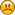So that will not work! Maybe some one else has a better way to do it.

####John Mac•69##### Re: can any one chech this code
« Reply #3 on: January 03, 2009, 07:27:05 AM »

####poppabear••2,235
• Briceville, TN, USA##### Re: can any one chech this code
« Reply #4 on: January 03, 2009, 10:18:49 AM »
Hey is what you need, this is from the "Conversational Wizard" I put up but the code in it will work for you, just modify it for your situation:

Sub Main()
'G68 angle calc
SetUserLed(1039,1)
x1=GetUserDRO(1081)
y1=GetUserDRO(1082)
x2=GetUserDRO(1095)
y2=GetUserDRO(1096)
D=1
pi = 4 * Atn(1)

If x2-x1=0 Then
Message("X2 cannot = X1!!")
Exit Sub
End If

If y2-y1=0 Then
Message("y2 cannot = y1!!")
Exit Sub
End If

If y2=0 Then
Message("y2 cannot = 0!!")
Exit Sub
End If

If x2=0 Then
Message("X2 cannot = 0!!")
Exit Sub
End If

If y2>y1 And x2>x1 Then
D=D*1
End If

If y2>y1 And x2<x1 Then
D=D*-1
End If

If y2<y1 And x2<x1 Then
D=D*1
End If

If y2<y1 And x2>x1 Then
D=D*-1
End If

a=y2-y1
b=x2-x1
ang=(180/pi) * Atn(a/b)

If D>0Then
SetUserDRO(1094,ang)
End If

If D<0Then
SetUserDRO(1094,ang)
End If

End Sub
Main

'scott
fun times

####swetha22•9##### Re: can any one chech this code
« Reply #5 on: January 03, 2009, 11:57:17 AM »
that's really nice and that's all i want ...thanks and thanks again.
swetha